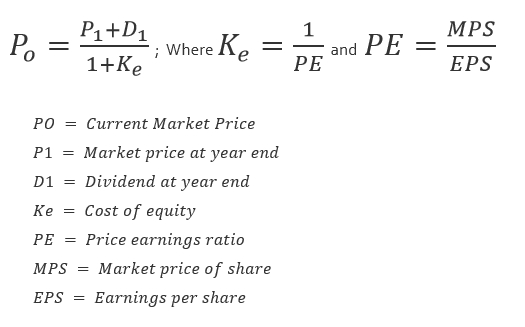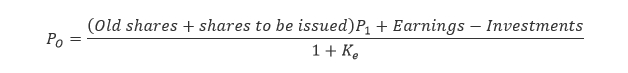# MM Theory of Capital Structure

What is Modigliani Miller Theory?

A theorem which states that –

• Market price of shares are unaffected,
• Irrespective of its dividend policy.

What are the assumptions?

• No taxes.
• No bankruptcy and agency cost.
• Symmetric information (investors and company having same information).
• Efficient market.

What is the formula?

####How to learn this?

• It is present value of future flow discounted at cost of equity (simple right!).
• In terms of layman, if you want to buy shares and you want to know the fair current market price (PO) and you know the dividend (D1) and Market price of share (P1) at year end, then discount it at (Ke).
• (Take the present value of whatever you will be having at year end i.e. dividend and share price)
• Discount it at Ke.

Note – Sometimes, you will be asked to find out the value of business then use this formula